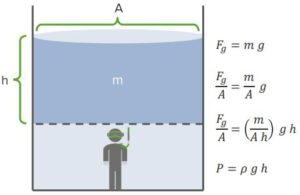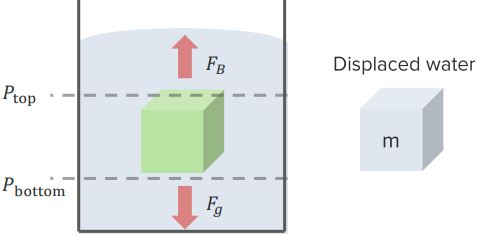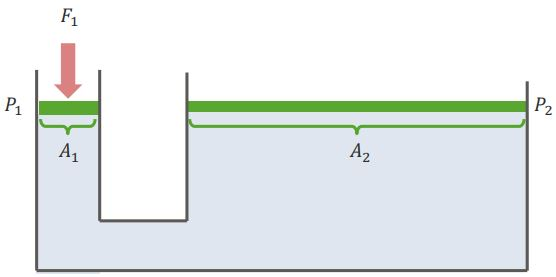Are you more of a visual learner? Check out our online video lectures and start your physics course now for free!Image: “Drop of water” by Tim Geers from the Netherlands – https://www.flickr.com/photos/timypenburg/4649617096/. License: CC BY-SA 2.0

Pressure

Definition

Pressure is defined as a continuous physical force that is exerted on or against an object by something that comes in contact with it. It can be described as the force per unit area. This concept is important in medicine, especially when dealing with blood flow (cardiovascular system) and with airflow (respiratory system).

Explanation

Imagine a beach ball full of air. The air inside keeps the ball inflated. Inside the ball, collisions take place against the wall of the ball that apply force. Only those collisions that are perpendicular to the wall have an effect. The air molecules moving along the sidewalls will not influence the balloon staying inflated.

Formula

The pressure formula is stated as P = F1 / A where P is the pressure, F1 is the force and A is the area involved. Looking at the units, we get:

(P) = kg / m-s2 = N / m2 = Pascals (Pa)

Pressure is measured in the Pascal units.

Atmospheric Pressure

When you are standing on Earth, pressure is exerted on you but you don’t feel it. This pressure is referred to as atmospheric pressure or Fair (the force that air exerts on a person). The reason you don’t feel atmospheric pressure is due to the water and air pressure inside of you pushing outwards. The balance of pressure doesn’t allow a person to feel the force of the air above. Atmospheric pressure can be measured in atmospheres or Pascals:

1 atm = 100,000 Pa = 100kPa

Hydrostatic Pressure“Hydrostatic-Pressure” Image created by Lecturio

When you go underwater, there are two weights acting on you from above: the atmospheric pressure and the water pressure. The water pressure is referred to as hydrostatic pressure or Gauge pressure. It is denoted as P = ρ g h, where ρ is the density of the liquid medium, g is the gravitational force, and h is the height of water above the person. So, the total pressure exerted on a person underwater is:

Ptotal = ρ g h + Patm

Archimedes’ Principle & Buoyancy

Definition

The famous Greek mathematician Archimedes stated that anybody, completely or partially submerged in a fluid at rest, is acted upon by an upward (buoyant) force. This force is equal to the weight of the fluid that the body displaces. Buoyancy occurs because the pressure of the water underneath is greater than the pressure of the water above. When measuring the displaced water, the calculation is based on the volume of the object that was submerged. The pressure acting upward is FB = buoyant force and the pressure acting downward is Fg = gravitational force.“Archimedes´-Principle” Image created by Lecturio

Flotation Rules

An object will float if:

FB > Fg

mwater g > mobject g

ρwater V > ρobject V

ρwater > ρobject

Thus buoyancy FB = mdisplaced water g

How much of the object will emerge from the water?

If an object is floating, it will emerge from the water until:

FB = Fg

mwater displaced = mobject

ρwater Vsubmerged = ρobject Vobject

Therefore, for floating objects: Vsubmerged / Vobject = ρobject / ρwater

Pascal’s Law

Definition

French scientist Blaise Pascal discovered an interesting phenomenon we now know as Pascal’s law or Pascal’s principle. It states that pressure is equally distributed throughout a fluid. In a fluid at rest in a closed container, a pressure change in one part is transmitted without loss to every part of the fluid and to the walls of the container.

Formula

In order to determine the pressures and forces associated with Pascal’s Law, the following must be observed:

Since the pressure is equally distributed throughout a fluid, P1 = P2.

Since pressure is related to force and area, then the following relationship occurs:

F1 / A1 = F2 / A2, thus there is equal force per unit area.“Pascal´s Law” Image created by Lecturio

Hydraulics

Pascal’s law is useful in machinery that uses hydraulics (the science involving the mechanical properties and uses of liquids). In these scenarios, one can gain a mechanical advantage by making one area greater than another, such that:

F2 = F1 (A2 / A1)

Ideal Hydrodynamics

Definition

Hydrodynamics is the branch of science concerned with forces acting on or exerted by fluids and the forces acting on solid bodies, immersed and in motion. Hydrodynamics contains six different parts: ideal flow, flow rate, continuity, Bernoulli’s principle, Venturi effect, and Pitot tube.

Ideal Flow

Ideal flow deals with an ideal fluid— one that is incompressible, not viscous, and laminar without turbulence. Liquids do not have the ability to be compressible, unlike gases. The flow in liquids occurs due to the lack of viscosity. The ideal flow must be laminar and orderly without turbulence or chaos. In general, laminar flow occurs at lower velocities and is smooth, not rough.

Flow Rate

The flow rate is the amount of fluid that flows at a given time. It is calculated as follows:

Flow rate = Volume per second

Q = Volume / Time = (Area x Distance) / Time

Thus, the flow Q = Area x Velocity (Q = A v) since velocity = distance / time

Continuity

When a fluid is in motion, it must move in such a way that mass is conserved. If the flow rate is too low, a vacuum situation will occur. If the flow rate is too high, overlap will occur.

Q1 = Q2 and A1 v1 = A2 v2

Bernoulli’s Principle

Daniel Bernoulli explained an important principle in fluid dynamics. His principle stated that an increase in the speed of a fluid occurs simultaneously with a decrease in pressure or a decrease in the fluid’s potential energy. At any portion along the tube, energy is conserved.

P1 + ½ ρ v12 + ρ g h1 = P2 + ½ ρ v22 + ρ g h2

The Venturi Effect

The Venturi effect is the reduction in fluid pressure that results when a fluid flows through a constricted section of a pipe. The constricted area will have a higher velocity but a lower pressure in a given system. In fluid dynamics, a fluid’s velocity must increase as it passes through a narrowing or constricted section of pipe  (according to the principle of mass continuity).

However, the fluid’s static pressure must decrease according to the principle of conservation of mechanical energy (Bernoulli’s principle, stated above). Thus, in a constricted section of pipe, fluid will have a higher velocity and lower pressure; and in a wider section of pipe, the fluid will have higher pressure and lower velocity.

Pitot Tube

A pitot tube is a pressure measuring instrument used to measure fluid flow velocity. The basic setup consists of a tube pointing directly into the fluid flow. As this tube contains fluid, pressure can be measured. The moving fluid is brought to rest (stagnates) as there is no outlet to allow flow to continue. This pressure is the stagnation pressure, or total pressure, of the fluid.

Using Bernoulli’s principle, we know that stagnation pressure = static pressure + dynamic pressure.

Ptotal = Pstatic + ½ ρ v2

Solving for velocity, v = √( (2 (Ptotal – Pstatic)) / ρ)

Learn. Apply. Retain.
Your path to achieve medical excellence.
Study for medical school and boards with Lecturio.